### General Physics, High School and College Freshman level### A Tutorial on The Basics of Refraction: Notes, Figures and Problems with Solutions

Target Audience: These notes might be of use to Indian students preparing for the ISC or CBSE Class 11 and 12 Examinations, IIT JEE (main and advanced), AIEEE; students from across the world preparing for their A Level Examinations, IB (International Baccalaureate) or AP Physics.

# (ii)    The ratio of the sines of the angle of incidence (i) and of the angle of refraction (r) is a constant quantity μ for two given media, which is called the refractive index of the second medium with respect to the first.

(sin i / sin r) = constant = μ

When light propagates through a series of layers of different medium as shown in the figure, then the Snell’s law may be written as

μ1 sin 1 = μ2 sin 2 = μ3 sin 3 =  μ4 sin 4 = constant

In general, μ sinθ  = constant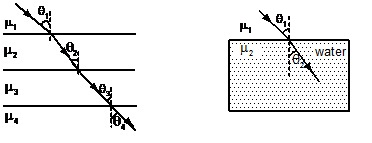Fig a (series of transparent layers of different refractive indices), Fig b (A light ray passing from air to water bends toward the normal)

When light passes from rarer to denser medium it bends toward the normal as shown in the fig.

According to Snell’s law

μ1 sin θ1 = μ2 sin θ2

When a light ray passes from denser to rarer medium it bends away from the normal as shown in the fig. b above

• This above formula can be applied at any interface effectively to find the deflection of light rays towards/away from the normal.

The image distance from the refracting surface is also known as Apparent depth or height.

Apparent Shift

Apparent shift = Object distance from refracting surface – image distance from refracting surface.

Δy (apparent shift) t = 1 - μ-1   where t is the object distance and  μ = μ1/ μ2

•    If there are a number of slabs with different refractive indices placed between the observer and the object.

Total apparent shift = ΣΔyi

### CRITICAL ANGLE AND TOTAL INTERNAL REFLECTION

Consider a ray of light that travels from a denser medium to a rarer medium. The angle of incidence for which the angle of refraction becomes 90? is called critical angle. This is used for constructing totally reflecting prisms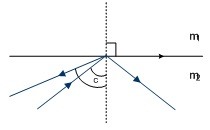Sin C / Sin 90o = 2m1 = m2 / m1

When the angle of incidence of a ray travelling from denser to rarer medium is greater then the critical angle, no refraction occurs. The incident ray is totally reflected back into the same medium. Here the laws of reflection hold good.

### THIN LENS FORMULA

For

u: object distance,

v: Image Distance

f: Focal length

We have 1/v-1/u=1/f.

Now, for R1 and R2 as the radii of curvature of 2 sides of a spherical lens, and n1, n2 being the refractive indices of the 2 sides respectively, we have the formula:

1/f=(n2/n1  -   1) * (1/R1 -  1/R2)

The above formula can be used for both concave and convex lens, the convention should be followed.

### POWER OF A LENS

The power of a lens can be quantitatively expressed as the reciprocal of the focal length of a lens. It is expressed in Dioptre(D). Power of a converging lens is said to be positive, as opposed to that of a diverging lens, which is negative. Also, the power of combination of 2 lenses in contact can be expressed as:

With 2 lenses with focal lengths f1, and f2 separated by distance d we have net Focal length as:

P = 1/f = 1/f1 + 1/f2 - d/(f1*f2) .

### PRISM

A prism has two plane surfaces AB and AC inclined to each other as shown in following  figure. A is called the angle of prism or refracting angle.

(i)    Refraction Through A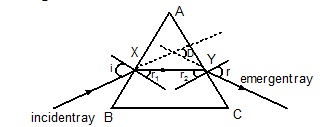A light ray striking at one face of a triangular glass prism gets refracted twice and emerges out from the other face as shown above. The angle between the emergent and the incident rays is called the angle of deviation (D). The angle between the two refracting faces involved is called the refracting angle (A) of the prism.

From AXY, we have:           A + (90° – r1) + (90° – r2) = 180°

As     r1 + r2 = A                                               (i)

Deviation D = (i – r1) + (e – r2)

D = (i + e) – (r1 + r2)

D = i + e – A

A + D = i + e                                      (ii)

We also have two equations from Snell's Law at X & Y.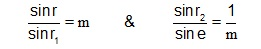### (i)     Angle of Deviation

It can be easily seen that if we reverse the emergent ray, it goes back along the same path. The angles of incidence and emergence get interchanged but the angle of deviation remains same.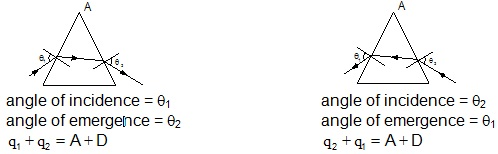Hence the same angle of deviation D is possible for two different angles of incidence :

θ1 and θ2, where θ1 + θ2 = A + D.

(ii)   Minimum Deviation

The angle of deviation is minimum when the path of light ray through the prism is symmetrical. i.e., angle of incidence = angle of emergence

(iii)       Grazing Incidence

When i = 90°, the incident ray grazes along the surface of the prism and the angle of refraction inside the prism becomes equal to the critical angle for glass - air. This is known as grazing incidence

(iv)       Grazing Emergence

When e = 90°, the emergent ray grazes along the prism surface. This happens when the light ray strikes the second face of the prism at the critical angle for glass - air. This is known as grazing emergence.

(v)       Maximum Deviation

The angle of deviation is same for both the above cases (grazing incidence & grazing emergence) and it is also the maximum possible deviation if the light ray is to emerge out from the other face without any total internal reflection.

(vi)     Dispersion of Light

When a ray of light passes through a prism, it splits up into rays of constituent colours or wavelengths. This phenomenon is called dispersion of light.

The refractive index of a medium is different for light rays of different wavelengths. Larger the wavelengths, the lesser is the refractive index.

i.e.    mred < mviolet because 1red > 1violet

A ray of white light passing through a prism gets splits into different colours because the deviation is more for violet and less for red. This phenomenon is known as dispersion.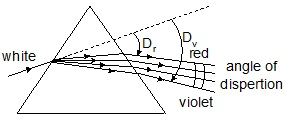For a prism with very small refracting angle A, the deviation D is given by:

d >> (m-1)A

Hence deviation of violet = Dv = (μv - 1)A,

and for red = Dr = (μr – 1)A

The angle between the red and violet rays is known as the angle of dispersion.

The angle of dispersion = f = Dv - Dr =  (mv - mr)A

This phenomenon arises due to the fact that refractive index varies with wavelength. It has been observed for a prism that μ decreases with the increase of wavelength, i.e.

mviolet > mred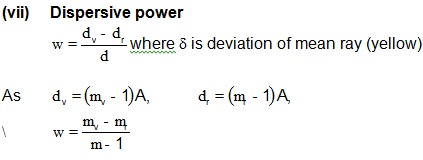### (viii)    Deviation without Dispersion

This mean an achromatic combination of two prisms in which net or resultant dispersion is zero and deviation is produced. For the two prisms,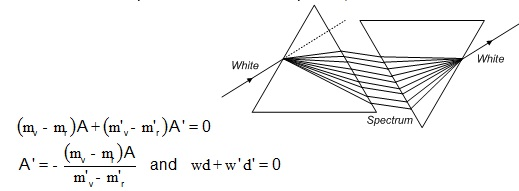Where w and w' are the dispersive powers of the two prisms and d and d' their mean deviations.

(ix)      Dispersion without deviation

A combination of two prisms in which deviation produced for the mean ray by the first prism is equal and opposite to that produced by the second prism is called a direct vision prism. This combination produces dispersion without deviation.

For deviation to be zero, (d + d') = 0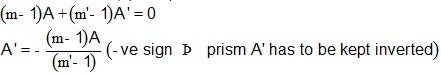The above concepts are imperative in solving problems related to this chapter.Do go through the tutorial problems and examples of this chapter.